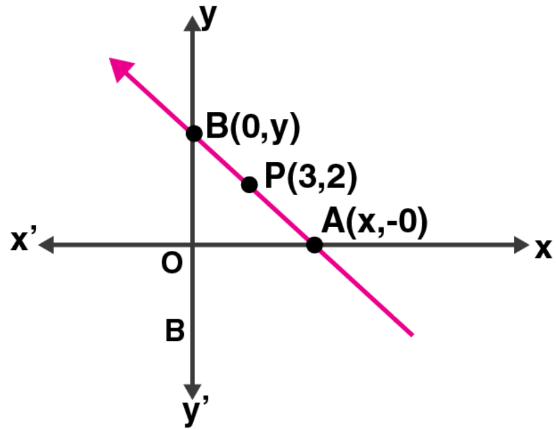Newbie

# Find the equation of the straight line containing the point (3, 2) and making positive equal intercepts on axes.

• -7

ICSE Board Question Based on Equation of a Straight Line Chapter of M.L Aggarwal for class10
In this question a straight line having a point and makes positive equal intercepts on axes.
You have to find the equation of the straight line.
This is the Question Number 28, Exercise 12.1 of M.L Aggarwal.

Share

1.Let the line containing the point P (3, 2) pass through x-axis at A (x, 0) and y-axis at B (0, y)Given, OA = OB

Thus, x = y

Now, the slope of the line (m) = y2 – y1/ x2 – x1

= 0 – y/ x – 0

= -x/x = -1

Hence, the equation of the line will be

y – y1 = m (x – x1)

y – 2 = -1 (x – 3)

y – 2 = -x + 3

⇒ x + y – 5 = 0

• 10